# 6

## Summary

### Factorization

The number 6 has the following factorization, with prime factors 2 and 3: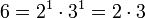### Properties and families

Property or family Parameter values First few members of the family Proof of satisfaction/membership/containment
perfect number: numbersuch that the divisor sum function ofisfirst perfect number divisor sum is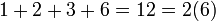primorial: product of the first few prime numbers product of the first two prime numbers (if we start the indexing from the zeroth primorial, which is an empty product and evaluates to 1, it is the third in the list) 1, 2, 6, 30, 210, 2310 View list on OEIS The first two primes are 2 and 3, and their product is 6.
factorial: product of the first few natural numbers product of the first three natural numbers (if we start the indexing from the zeroth factorial, which is an empty product and evaluates to 1, it is the fourth in the list) 1, 2, 6, 24, 120, 720, 5040, 40320, 362880, [SHOW MORE] View list on OEISlcm of all numbers so far: lcm of the first few natural numbers lcm of the first three natural numbers 1, 1, 2, 6, 12, 60, 60, 420, [SHOW MORE] View list on OEIS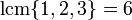.

## Arithmetic functions

Function Value Explanation
Euler totient function 2 The Euler totient function is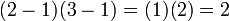.
universal exponent 2 The universal exponent is.
divisor count function 4where the first 1s in both factors are the multiplicities of the prime divisors.
divisor sum function 12times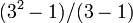equals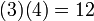.
Mobius function 1 The number is square-free and has an even number of prime divisors (2 prime divisors).